Courses

# Hots Questions- Surface Areas and Volumes Class 9 Notes | EduRev

## Mathematics (Maths) Class 9

Created by: Full Circle

## Class 9 : Hots Questions- Surface Areas and Volumes Class 9 Notes | EduRev

The document Hots Questions- Surface Areas and Volumes Class 9 Notes | EduRev is a part of the Class 9 Course Mathematics (Maths) Class 9.
All you need of Class 9 at this link: Class 9

Question 1. A brick measures 30 cm × 10 cm ×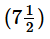cm. How many bricks will be required for a wall 30 m long, 2 m high and (3/4)m thick?

Hint: Volume of wall (cuboid) = 30 × 2 × (3/4) m= 45 m3
Volume of 1 brick  =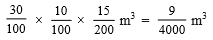∴ Required number of bricks =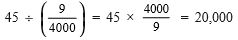Question 2. The surface area of a cube is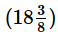m2. Find its volume.
Hint:
Total S.A. = 6(side)2 ⇒ side2 =÷ 6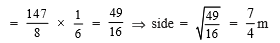∴ Volume = (side)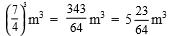Question 3. A hemispherical tank is emptied by a pipe at the rate of 5 litres per minute. How long will it take to half empty the tank if it is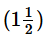metre in diameter. [Take π = (22/7) and 1l = 1000 cm]3

Hint: Radius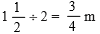Volume of the hemisphere = (1/2) (volume of a sphere) =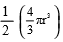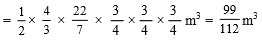Volume to be emptied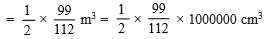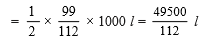∵ 5 litres is emptied in 1 minute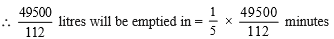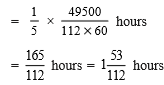,

,

,

,

,

,

,

,

,

,

,

,

,

,

,

,

,

,

,

,

,

;